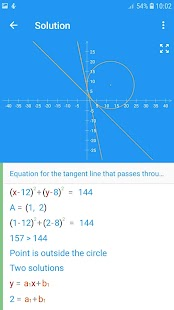# Math Studio v2.33 build 96 [Paid] [Latest]

Report this app

## DescriptionAll in one. Step by step solution.
– Geometry
– Linear Function
– Linear System
– Math Sequences
– Algebra
– Vectors (new!!!!)

Languages: English, Deutsch, español, français, italiano, polski, português, русский, Türkçe, Nederlands

Math Studio is advanced application for solving math problems. There is a complete solution delivered for each issue to satisfy every teacher or student.
Additionally, it contains all the useful formulas.

GEOMETRY
– square
– rectangle
– rhombus
– parallelogram
– triangle
– equilateral triangle
– right triangle
– isosceles triangle
– circle
– annulus
– trapezoid
– right trapezoid
– isosceles trapezoid,
– regular hexagon
– sphere
– cylinder
– cone
– regular tetrahedron
– cube
– square prism
– cuboid
– ellipse
– regular pentagon
– kite
– square pyramid
– triangular pyramid
– thales’ theorem
– triangular prism
– regular triangular prism
– trigonometry
– regular octagon
– regular dodecagon
– hexagonal prism
– hexagonal pyramid
– truncated cone
– pentagonal prism

– standard form
– vertex form
– factored form
– discriminant of the quadratic function
– real roots (zeros)
– vertex of a parabola
– intersection of the Y-Axis
– monotonicity (increasing, decreasing)
– positive and negative values (inequalities)

LINEAR FUNCTION
– slope-intercept form
– standard form
– distance between two points
– midpoint of a line segment
– line segment bisector
– parallel line
– perpendicular line
– distance from a point to a line
– equation of the line passing through 2 points

LINEAR SYSTEM
Four methods for solving systems:
– Substitution method
– Elimination method
– Graph method
– Method of determinants

MATH SEQUENCES
– properties of geometric progression: initial term, any mth term and nth term, ratio, sum of n terms, general formula
– properties of arithmetic progression: initial term, any mth term and nth term, difference, sum of n terms, general formula
– properties of geometric series: initial term, ratio, sum

ALGEBRA
– greatest common divisor (gcd)
– least common multiple (lcm)

What’s New:
2.33
– Analytic geometry
– Circle with center and point
– Circle with center and radius
– Circle with three points

Screenshots

••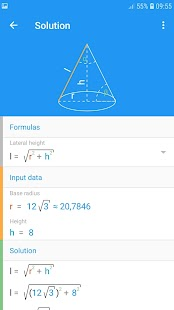•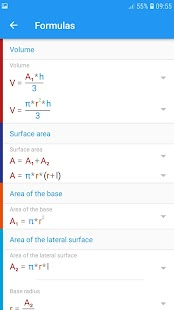•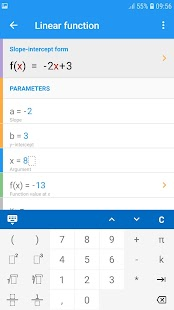•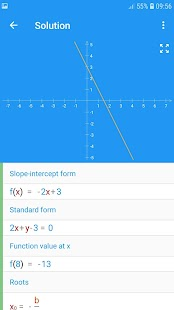••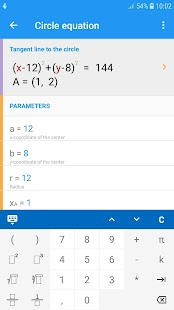•